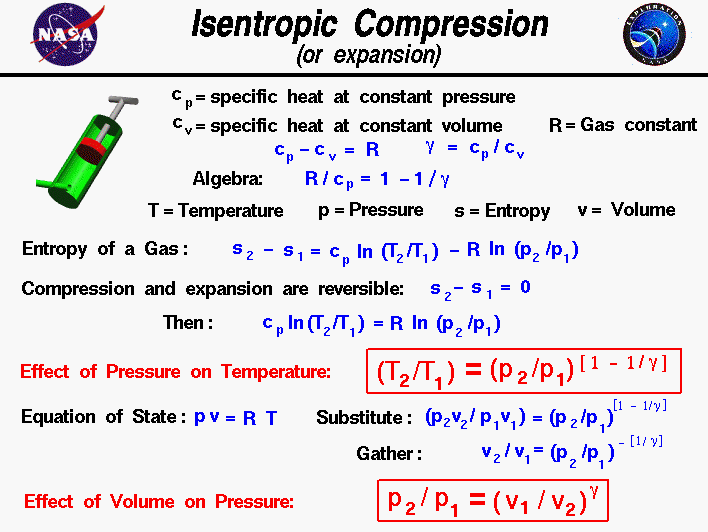+ Text Only Site
+ Non-Flash Version
+ Contact GlennThermodynamics is a branch of physics which deals with the energy and work of a system. Thermodynamics deals only with the large scale response of a system which we can observe and measure in experiments. In rocket science, we are most interested in thermodynamics for the role it plays in engine design and high speed flows. On this slide we derive two important equations which relate the pressure, temperature, and volume which a gas occupies during reversible compression or expansion. Such a process occurs in the launch of a compressed air rocket or of a water rocket. A pump, shown in green on the figure, is used to increase the pressure of the rocket. As the red piston moves in the cylinder, the volume of the gas inside the cylinder is changed. This change in volume results in a change in pressure and temperature of the gas which determines how much work the piston can deliver. During the motion of the piston, we are going to assume that no heat is transferred into the cylinder. We are further going to neglect any friction between the piston and cylinder and assume that there are no energy losses of any kind. (In reality there are small losses and we account for the losses by an "efficiency factor" applied to the result we obtain assuming no losses.) The resulting compression and expansion are reversible processes in which the entropy of the system remains constant. We can use equations for the entropy to relate the flow variables of the system. We begin our derivation by determining the value of a factor which we will need later. From the definitions of the specific heat coefficients, the specific heat at constant pressure cp minus the specific heat at constant volume cv is equal to the gas constant R: cp - cv = R and we define the ratio of specific heats to be a number which we will call "gamma" gamma = cp / cv If we divide the first equation by cp, and use the definition of "gamma" we obtain: R / cp = 1 - (1 / gamma) = (gamma - 1) / gamma Now we use the equation we have derived for the entropy of a gas: s2 - s1 = cp ln(T2 / T1) - R ln(p2 / p1) where the numbers 1 and 2 denote the states at the beginning and end of the compression process, s is the entropy, T is the temperature, p is the pressure, and "ln" denotes the natural logarithm function. Since there is no heat transferred into the cylinder and no other losses, the change in entropy is zero. Then the equation becomes: cp ln(T2 / T1) = R ln(p2 / p1) We divide both sides by "cp" and take the exponential function of both sides (this "un-does" the logarithms). T2 / T1 = (p2 / p1) ^ (R / cp) where the symbol "^" denotes an exponent. Now we substitute the expression for "R / cp" to obtain: T2 / T1 = (p2 / p1) ^ [(gamma - 1)/gamma] During the compression process, as the pressure is increased from p1 to p2, the temperature increases from T1 to T2 according to this exponential equation. "Gamma" is just a number that depends on the gas. For air, at standard conditions, it is 1.4. The value of (1 - 1/gamma) is about .286. So if the pressure doubled, the temperature ratio is 1.219. The key point here is that we have a function that relates the temperature change to the pressure change during a compression process. We can use the equation of state to derive the relation between the volume change and the pressure change. The equation of state is: p * v = R * T where v is the specific volume occupied by the gas. If we substitute this expression for T into the temperature equation, we obtain: (p2 * v2) / (p1 * v1) = (p2 / p1) ^ [(gamma - 1)/gamma] Multiply both sides by (p1 / p2) to get: v2 / v1 = (p2 / p1) ^ ( - 1/gamma) p2 / p1 = (v1 / v2) ^ (gamma) The quantity (v1 / v2) is the ratio of the volume at state 1 and state 2 and is called the compression ratio. For v2 less than v1, the pressure p2 is greater than p1. With this equation we can determine the change in pressure for a given compression ratio. And using the previous equation we know the change in temperature as well. The value of the compression ratio is a function of the design of the pump used to launch our rockets. Guided ToursIsentropic Flow:Activities: Related Sites: Rocket Index Rocket Home Beginner's Guide Home+ Inspector General Hotline + Equal Employment Opportunity Data Posted Pursuant to the No Fear Act + Budgets, Strategic Plans and Accountability Reports + Freedom of Information Act + The President's Management Agenda + NASA Privacy Statement, Disclaimer, and Accessibility CertificationEditor: Tom Benson NASA Official: Tom Benson Last Updated: May 13 2021 + Contact Glenn